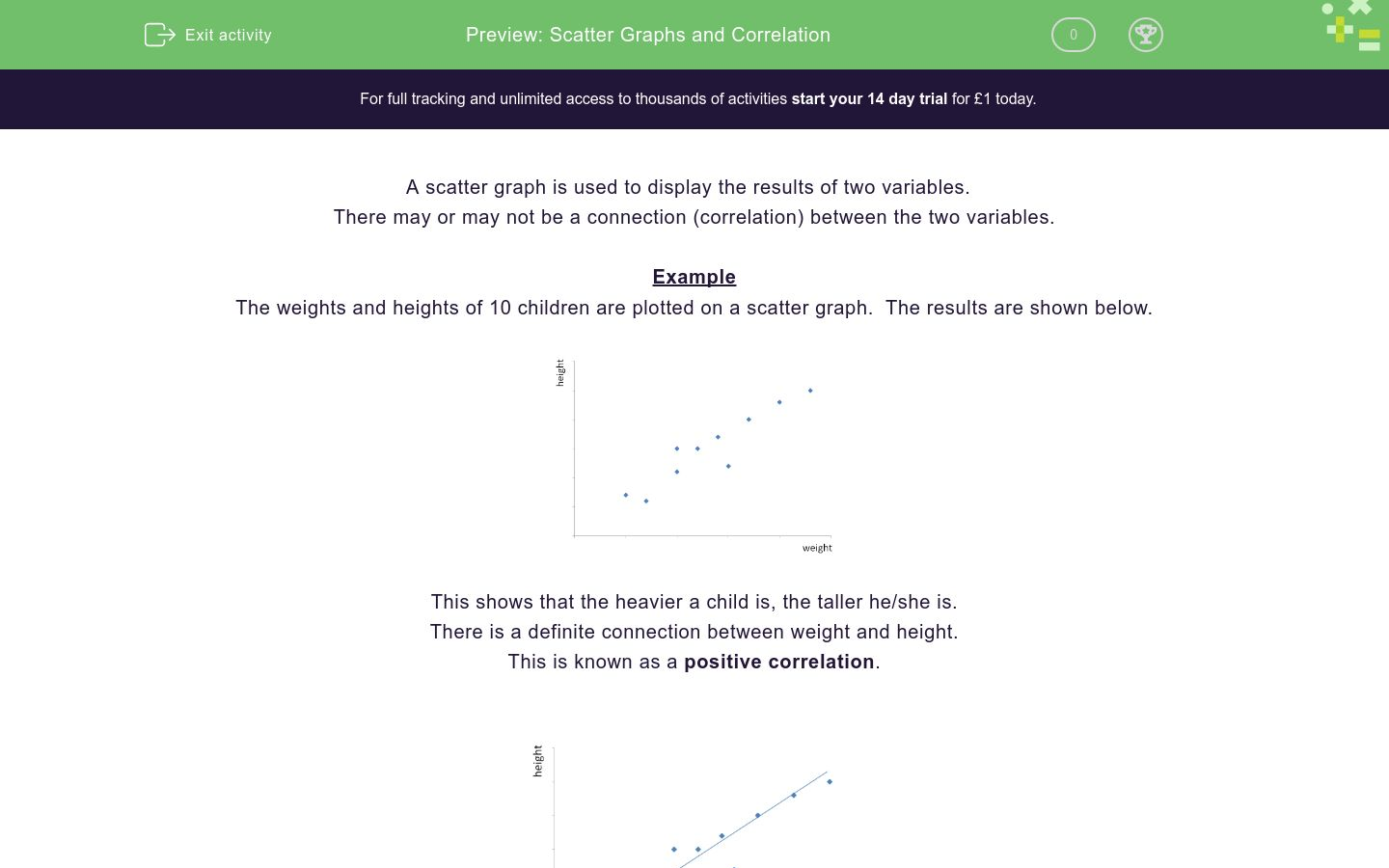# Scatter Graphs and Correlation

In this worksheet, children must interpret information shown on a scatter graph and understand the term correlation.Key stage:  KS 3

Curriculum topic:   Statistics

Curriculum subtopic:   Understand the Relationship Between Two Variables

Difficulty level:### QUESTION 1 of 10

A scatter graph is used to display the results of two variables.

There may or may not be a connection (correlation) between the two variables.

Example

The weights and heights of 10 children are plotted on a scatter graph.  The results are shown below.This shows that the heavier a child is, the taller he/she is.

There is a definite connection between weight and height.

This is known as a positive correlation.We can draw a line that best fits this data.  It goes in the direction of the plotted points and has roughly the same number of points above it as below.

It is known as the line of best fit.

The following scatter graphs show negative correlation and no correlation.negative correlation                     no correlation

Which type of correlation is shown in the following graph?positive

negative

no

Which type of correlation is shown in the following graph?positive

negative

no

Which type of correlation is shown in the following graph?positive

negative

no

Which graph best shows the connection between the age of a car and its mileage?A                               B                                  C

A

B

C

Which graph best shows the connection between the distance travelled on a car journey and the amount of petrol left in the tank?A                               B                                  C

A

B

C

Which graph best shows the connection between the age of a normal car and its value?A                               B                                  C

A

B

C

Which graph best shows the height of a child and their mark in a maths exam?A                               B                                  C

A

B

C

Which graph best shows the outside temperature and the number of ice creams sold?A                               B                                  C

A

B

C

Which line is the line of best fit?A

B

C

Which line is the line of best fit?A

B

C

• Question 1

Which type of correlation is shown in the following graph?negative
• Question 2

Which type of correlation is shown in the following graph?no
• Question 3

Which type of correlation is shown in the following graph?positive
• Question 4

Which graph best shows the connection between the age of a car and its mileage?A                               B                                  C

A
EDDIE SAYS
As a car gets older, it's mileage goes up.
• Question 5

Which graph best shows the connection between the distance travelled on a car journey and the amount of petrol left in the tank?A                               B                                  C

B
EDDIE SAYS
As a car travels further on a journey, the amount of petrol in its tank goes down.
• Question 6

Which graph best shows the connection between the age of a normal car and its value?A                               B                                  C

B
EDDIE SAYS
Usually, as a car gets older, it's value goes down.
• Question 7

Which graph best shows the height of a child and their mark in a maths exam?A                               B                                  C

C
EDDIE SAYS
There is no correlation between a child's height and how well they do in a maths test.
• Question 8

Which graph best shows the outside temperature and the number of ice creams sold?A                               B                                  C

A
EDDIE SAYS
Usually, the warmer it is outside, the more ice creams are sold.
• Question 9

Which line is the line of best fit?B
• Question 10

Which line is the line of best fit?C
---- OR ----

Sign up for a £1 trial so you can track and measure your child's progress on this activity.

### What is EdPlace?

We're your National Curriculum aligned online education content provider helping each child succeed in English, maths and science from year 1 to GCSE. With an EdPlace account you’ll be able to track and measure progress, helping each child achieve their best. We build confidence and attainment by personalising each child’s learning at a level that suits them.

Get started